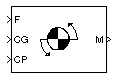Moments about CG due to Forces

Compute moments about center of gravity due to forces applied at a point, not center of gravity

• Library:
• Aerospace Blockset / Mass Properties

•Description

The Moments about CG due to Forces block computes moments about center of gravity due to forces that are applied at point CP, not at the center of gravity.

Ports

Input

expand all

Forces applied at point CP, specified as a three-element vector.

Data Types: double

Center of gravity, specified as three-element vector.

Data Types: double

Application point of forces, specified as a three-element vector.

Data Types: double | bus

Output

expand all

Moments at the center of gravity in x-axis, y-axis and z-axis, returned as a three-element vector.

Data Types: double

Extended Capabilities

C/C++ Code GenerationGenerate C and C++ code using Simulink® Coder™.